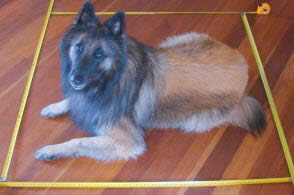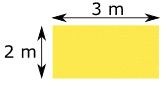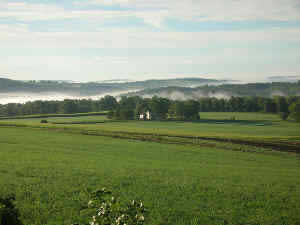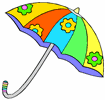# Metric Area

These are the most common measurements of area (from smallest to largest):

• Square Millimeter
• Square Centimeter
• Square Meter
• Hectare
• Square KilometerAriel the Dog is waiting patiently
inside 1 square meter.

### Square Meter

The square meter is the basic unit of area of the Metric System.

Area is length by length, so:

A square that is 1 meter on each side
is 1 square meter.

The Unit is meters × meters, which is written m2 (square meters).

You could have other shapes (such as a rectangle that is ½ a meter by 2 meters) that also make 1 square meter.

### Example: How big is this rectangle?It is 2 meters by 3 meters, so it is 2 m × 3 m = 6 m2   (6 square meters)

### Square Meter vs Meter Square

Be careful to say "square meters" not "meters squared":### Square MillimeterA square millimeter is millimeters × millimeters, which is written mm2.

A millimeter is one-thousandth of a meter (1/1000 meter), so a square millimeter is also:

11000 × 11000  =  11,000,000 of a square meter

In other words a square millimeter is one-millionth of a square meter.

So a square meter has 1,000,000 square millimeters.

### Square CentimeterA square centimeter is centimeter × centimeter,

It is written cm2

A centimeter is one-hundredth of a meter (1/100 meter), so a square centimeter is also:

1 100 × 1 100   =   1 10,000 of a square meter

In other words a square centimeter is one-ten-thousandth of a square meter.

So a square meter has 10,000 square centimeters.### Hectare

A hectare (ha) is an area equal to a square that is 100 meters on each side.

So a hectare has 100 m × 100 m = 10,000 m2 (square meters).

Hectares are commonly used to measure land.

### Square Kilometer

A square kilometer is kilometer × kilometer, which is written km2.

A kilometer is a thousand meters, so a square kilometer is also:

1,000 m × 1,000 m = 1,000,000 m2 (square meters)

In other words a square kilometer is one-million square meters.

Square kilometers are commonly used to measure large areas of land.

## Some Examples

A square meter is:

• about half the area of a doorway
• the area of an A0 sheet of paper (or 16 sheets of A4 paper)about the area covered by an opened large umbrella